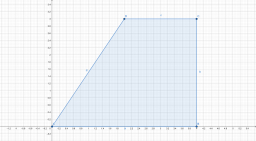# Coordinates 66474

Draw a trapezoid in the coordinate system with bases 4cm long, 2cm long, and 3cm high. Please write down the coordinates of its vertices.

x =  1

### Step-by-step explanation:Did you find an error or inaccuracy? Feel free to write us. Thank you!

Tips to related online calculators
Line slope calculator is helpful for basic calculations in analytic geometry. The coordinates of two points in the plane calculate slope, normal and parametric line equation(s), slope, directional angle, direction vector, the length of the segment, intersections of the coordinate axes, etc.

#### Level of the problem:

We encourage you to watch this tutorial video on this math problem: